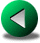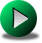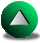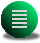Previous: Gravity Waves in a
Next: Conservative Properties of the
Up: Testing the Different Numerical

# The Wind-driven Circulation in a Circular Domain

A linear analytical solution can be found for the wind-driven problem in a circular domain with Coriolis forces and damped by a linear bottom friction. No viscosity is included. The boundary condition is simply the no-normal flow condition at the model boundary. The steady state linearized shallow water equations in cylindrical coordinates for this problem are(3.6)

where the wind forcing is given in cylindrical coordinates by the relationship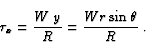(3.7)

where R is the radius of the circular domain. From (3.6-3.8), we derive an equation for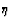(3.8)

with the boundary condition of no-normal flow(3.9)

This leads to the solution without Coriolis force,(3.10)

and with Coriolis force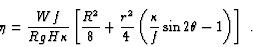(3.11)

With or without the Coriolis terms, the velocity components take the simple form of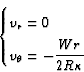(3.12)

which translate in the Cartesian coordinate system to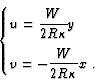(3.13)

We perform a one year spin-up for all models with W=10-4m2s-2, f=10-4s-1 or zero and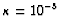s-1. This is enough to converge to a steady state accurate at six digits for the kinetic energy. The normalized error is computed in the same manner as in (3.5) but using the elevation field. We focus on the elevation this time because, for the HT and LLS FE models, the pressure basis functions are different from the basis functions used to represent the velocity. Furthermore, the previous test case does not allow for an interesting comparison of the elevation fields (the elevation is imposed at initial time), whereas this one does.

We first analyze the results from the C-grid model. Because of the presence of steps (Fig. 3.8), it is not clear which opposing effect is dominant when the resolution is increased: an increased accuracy in the interior and a more accurate representation of the boundary, or a lower accuracy because of the increased number of steps. For brevity, we only show the results for one case, at f=0, since convergence properties are not significantly different than those at. Figure 3.9 shows the convergence of the normalized error inwith increasing resolution. It appears that the convergence order of the C-grid FD model is closer to one (1.1 when f=0 and 1.3 when f=10-4s-1) than two, the maximum for this second order FD formulation. Therefore, the steps have a direct influence on the order of the FD model. The order is reduced compared to the previous test-case with straight walls. The perturbation due to the singular steps on the flow does degrade the accuracy, although not to the point that the errors increases with increasing resolution.We now compare the solution from the C-grid FD model with the O-FDM4 and R-FDM4 models. Figure 3.9 shows that the order of the A-grid model is actually less than two in presence of step-like walls. Furthermore, there is no longer a difference, in term of truncation order, between the second order C-grid and the 4th order A-grid models --unlike the case with straight walls. Therefore, the presence of steps along irregular boundaries has a detrimental effect on the accuracy of high order FD formulations if the flow is allowed to slip along the walls.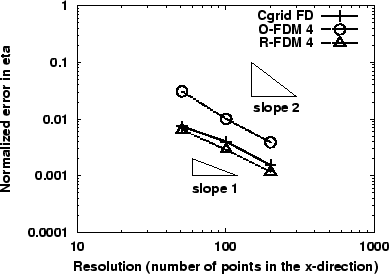We now compare the FE models to the C-grid model. In this circular geometry, all FE models have the advantage that the representation of the boundary is improving as the resolution is increased. Therefore, it should be possible to observe convergence order close or even exceeding two. Figure 3.10 and Table 3.2 show that all FE models have a convergence rate close to second order except for the LLS model. The LLS model also shows the largest errors. The reasons for the poor performance of this model are as follows. Firstly, the geometry is resolved by the macro-elements. Thus the representation of the boundary suffers from being half sampled compared to the permitted resolution. Second, we focus here on the elevation errors which are always larger for the LLS model because the piecewise constant basis functions are not as accurate as those of the other models. For the HT model, the improvement in the error compared to the previous test-case is probably due to the basis function forbeing continuous. In fact, all FE models used this basis function for the elevation except for the LLS model. Hence in terms of accuracy, all FE models appear to perform better than FD models in non-rectangular geometries for linear problems, except for the LLS model. In terms of cost, the equal-order FE models are the most effective. However, we still need to demonstrate the efficiency of FE models for nonlinear problems before concluding on the general effectiveness of FE models in irregular domains.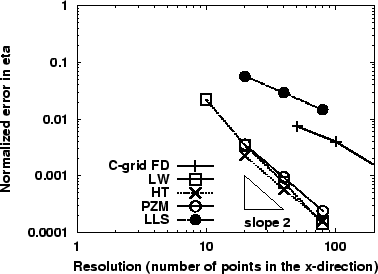For the SE model, the results are given in Fig. 3.11 where we compare the solutions from the C-grid FD, LW FE and SE models. The results for the SE model shows a surprising feature. The 3rd order SE model has a better accuracy than the FE model but the errors for the 5th and 7th order SE are larger than expected. The convergence order is also affected (see Table 3.2). In this particular example, the main source of errors comes from the discretization of the circular geometry by piecewise parabolas. A quadratic spline description of the circular boundary allows for (at least) a 3rd convergence order. This explains why the convergence order for the 3rd order SE model appears optimal but less optimal for the 5th and 7th order SE model. The order of the solution improves in the interior but the error along the boundary being larger leads and causes a overall loss in the convergence order. One solution would be to implement more complex curved elements along the boundary (using cubic or more splines), but as explained in Section 2.4.4, increasing the order of the piecewise curves along the curved elements is not always practical.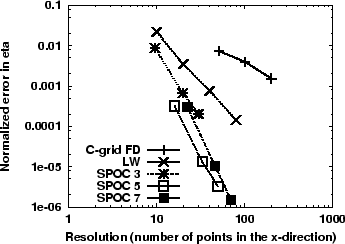Table 3.2: Convergence order in elevation, for the different models for the linear wind-driven experiment in a circular domain without Coriolis terms.
 Model convergence order for the error inC-grid FD 1.15 O-FDM 4 1.51 R-FDM 4 1.24 LW 2.40 HT 1.91 LLS 0.98 PZM 1.94 SPOC 3 3.33 SPOC 5 4.09 SPOC 7 4.64Previous: Gravity Waves in a
Next: Conservative Properties of the
Up: Testing the Different Numerical

Frederic Dupont
2001-09-11